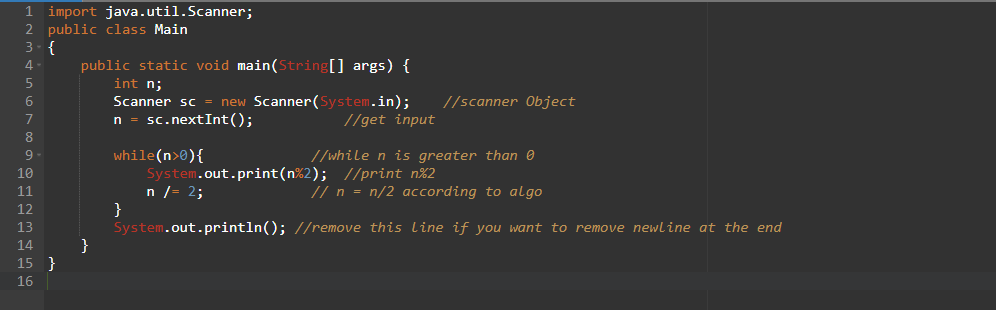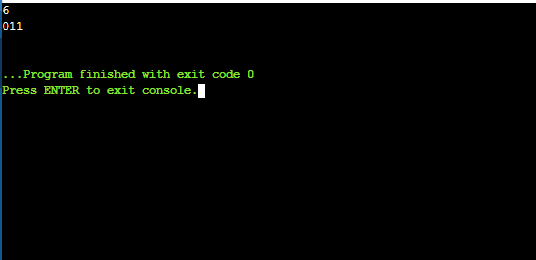##### Question

In: Computer Science

# Write a program that takes in a positive integer as input, and outputs a string of 1's and 0's representing the integer in binary. For an integer x, the algorithm is:

In Java

Write a program that takes in a positive integer as input, and outputs a string of 1's and 0's representing the integer in binary. For an integer x, the algorithm is:

As long as x is greater than 0
Output x % 2 (remainder is either 0 or 1)
x = x / 2

Note: The above algorithm outputs the 0's and 1's in reverse order.

Ex: If the input is:

6

the output is:

011

6 in binary is 110; the algorithm outputs the bits in reverse.

## Solutions

##### Expert Solution

Main.java

import java.util.Scanner;
public class Main
{
public static void main(String[] args) {
int n;
Scanner sc = new Scanner(System.in); //scanner Object
n = sc.nextInt(); //get input

while(n>0){ //while n is greater than 0
System.out.print(n%2); //print n%2
n /= 2; // n = n/2 according to algo
}
System.out.println(); //remove this line if you want to remove newline at the end
}
}

Screenshot of the code:Output:## Related Solutions

##### Write a program that takes in a positive integer as input, and outputs a string of 1's and 0's representing the integer in binary. For an integer x, the algorithm is:
USE Coral Write a program that takes in a positive integer as input, and outputs a string of 1's and 0's representing the integer in binary. For an integer x, the algorithm is:As long as x is greater than 0    Output x % 2 (remainder is either 0 or 1)    x = x / 2Note: The above algorithm outputs the 0's and 1's in reverse order.Ex: If the input is 6, the output is:011(6 in binary is 110; the algorithm outputs the bits in reverse).
##### [C] Write a program that takes in a positive integer as input, and outputs a string of 1's and 0's representing the integer in binary. For an integer x, the algorithm is:
6.19 LAB: Convert to binary - functionsWrite a program that takes in a positive integer as input, and outputs a string of 1's and 0's representing the integer in binary. For an integer x, the algorithm is:As long as x is greater than 0    Output x % 2 (remainder is either 0 or 1)    x = x / 2Note: The above algorithm outputs the 0's and 1's in reverse order. You will need to write a second function to reverse the string.Ex: If the input is:6the output is:110Your program must define and call the following two functions. The IntegerToReverseBinary function...
##### (Python) Write a program that takes in a positive integer as input, and outputs a string of 1's and 0's representing the integer in binary. For an integer x, the algorithm is:
In Python8.21 Program: Convert to binary. Sections 2.7, 3.8, 5.2. Functions.Write a program that takes in a positive integer as input, and outputs a string of 1's and 0's representing the integer in binary. For an integer x, the algorithm is:As long as x is greater than 0    Output x % 2 (remainder is either 0 or 1)    x = x // 2Note: The above algorithm outputs the 0's and 1's in reverse order. You will need to write a second function to reverse the string.Your program must define and call the following two functions. The function integer_to_reverse_binary() should return...
##### rogram that takes in a positive integer as input, and outputs a string of 1's and 0's representing the integer in binary.
Write a program that takes in a positive integer as input, and outputs a string of 1's and 0's representing the integer in binary. For an integer x, the algorithm is:As long as x is greater than 0    Output x % 2 (remainder is either 0 or 1)    x = x // 2Note: The above algorithm outputs the 0's and 1's in reverse order. You will need to write a second function to reverse the string.Ex: If the input is:6the output is:110Your program must define and call the following two functions. The function integer_to_reverse_binary() should return a string of 1's...
##### Write a program that takes in a positive integer as input, and outputs a string of...
Write a program that takes in a positive integer as input, and outputs a string of 1's and 0's representing the integer in binary. For an integer x, the algorithm is: As long as x is greater than 0 Output x % 2 (remainder is either 0 or 1) x = x / 2 Note: The above algorithm outputs the 0's and 1's in reverse order. Ex: If the input is: 6 the output is: 011 6 in binary is...
##### C++ Write a program that takes a string and integer as input, and outputs a sentence...
C++ Write a program that takes a string and integer as input, and outputs a sentence using those items as below. The program repeats until the input string is "quit". If the input is: apples 5 shoes 2 quit 0 the output is: Eating 5 apples a day keeps your doctor away. Eating 2 shoes a day keeps your doctor away.
##### USING C# 1. Write a program that takes outputs a string, an integer and a floating-point...
USING C# 1. Write a program that takes outputs a string, an integer and a floating-point number separated by commas. Sample output: Bob Marley, 20, 5.2 2. 2. Write a program that asks the user for a string of letters of any size (no spaces), and finally a special character (values 33 to 47 in the Ascii table, or ‘!’ to ‘/’). Generate a random number of any size, integer or floating point, and combine those three pieces of information...
##### Write a function decimalToBinary(n) that converts a positive decimal integer n to a string representing the...
Write a function decimalToBinary(n) that converts a positive decimal integer n to a string representing the corresponding binary number. Do the conversion by repeatedly dividing the number n by 2 using integer division, keepting track of the remainders, until the number is reduced to 0. The remainders written in reverse order form the binary number string. Do integer division of 5 by 2, so that N//2 is 2 with remainder 1. Now divide 2 by 2 to get 1 with...
##### a program that takes an integer and outputs the resulting factorial number.
assembly x86 language program a program that takes an integer and outputs the resulting factorial number.
##### Matlab Create/write a function that takes an input of x and y data, and a string...
Matlab Create/write a function that takes an input of x and y data, and a string (either linear? or quadratic), sets up a linear system of equations (Ax = b), and solves and plots the model.